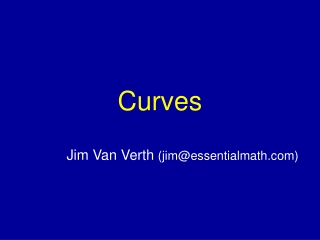DownloadDownload PresentationCurves

# Curves

Télécharger la présentation## Curves

- - - - - - - - - - - - - - - - - - - - - - - - - - - E N D - - - - - - - - - - - - - - - - - - - - - - - - - - -
##### Presentation Transcript

1. Curves Jim Van Verth (jim@essentialmath.com)

2. Animation • Problem: want to replay stored set of transformations • Generated by artist • Generated by motion capture • Two main issues • Replaying position • Replaying orientation Essential Math for Games

3. Animation of Position • Set of points representing position • Three issues to handle • Frame rate higher than sample rate • Reduce number of samples to save memory • Blend between two animation sets Essential Math for Games

4. Animation of Position • Idea: use set of points to define a function • Can then sample points along that function to find new positions • Two possibilities: • Interpolation (passes through points) • Approximation (passes near points) Essential Math for Games

5. Parametric Curve • Function Q(t) • Maps parameter t to point on curve Q • Commonly broken into ( x(t), y(t), z(t) ) • Called space curve • Derivative is tangent ( x'(t), y'(t), z'(t) ) Essential Math for Games

6. Linear Interpolation • Two points define a line • Can generate new points between them Essential Math for Games

7. Linear Interpolation • Do this using parameterization of line • Q(t) = P0 + t (P1 – P0)= (1 –t) P0 + t P1 • Parameter tcontrols position on line • Curve only defined where t in [0,1] P1 P0 Essential Math for Games

8. Multiple Points • Can linearly interpolate from point to point (piecewise linear curve) • Problem: get discontinuity at each point • I.e. not smooth Demo Essential Math for Games

9. Continuity • Continuous function has no ‘gaps’ • C-continuity • Number indicates derivative continuity • I.e. C1 means 1st derivative continuous • Tangents equal at joins • G-continuity • Tangents point in same direction Essential Math for Games

10. Hermite Curves • One solution • Idea: Cubic curve between each point • Tangents at each point specify how curve should go • Piecewise Hermite spline Demo Essential Math for Games

11. Hermite Curves • For points P0,P1 and tangents P'0,P'1 function is: P'1 P'0 P1 P0 Essential Math for Games

12. Hermite Curves • At each sample, two tangents – in and out • For smooth curve (C1) • Make them equal • Smooth curve but change in speed (G1) • Same direction, different lengths • For crease (C0) • Different directions Essential Math for Games

13. Computing Tangents • Export from commercial tools • May have to tweak to guarantee results • Export from homemade tools • Clamped, natural, cyclic, acyclic splines • Maintain C2 continuity • Solution to system of linear equations Essential Math for Games

14. Computing Tangents • Catmull-Rom spline • Tangent half vector between neighboring points • Tangent undefined at end points Pi Pi+1 Pi-1 Essential Math for Games

15. Bezier Curve • Instead of two tangents, have two approximating control points • Curve doesn’t pass through control points • Their position controls shape of curve • Curve lies in convex hull Essential Math for Games

16. Bezier Curve • For points P0,P1, P2, P3 function is: P2 P0 P3 P1 Essential Math for Games

17. Bezier Curve • Control points provide good user interface • Align control points between curve segments for continuity Essential Math for Games

18. De Castlejau's method • Fast Bezier subdivision • Find percentage along each segment, then along resulting segments • Result is control pts for two subcurves P2 P1 P3 P0 Essential Math for Games

19. De Castlejau’s Method • Useful for rendering • Subdivide until only “straight” subcurves • Straight if P1 & P2 are close to P0P3 • Useful for measuring length • Subdivide until ||P0P1|| + ||P1P2|| + ||P2P3|| is close to ||P0P3|| • Add up all the sublengths Essential Math for Games

20. B-splines • Generally curve approximates points, i.e. doesn’t pass through them • Continuous through 2nd derivative • Provides local control Essential Math for Games

21. NURBS • Non-Uniform Rational B-Splines • Non-uniform = curve segments have unequal spacing by parameter • Rational = can apply weights to sample points (good for conic sections) • Result: very useful, but not cheap • Mostly used for parametric surfaces Essential Math for Games

22. References • Parent, Rick, Computer Animation: Algorithms and Techniques, Morgan Kaufmann, 2002. • Bartels, Beatty, Barsky, An Introduction to Splines for use in Computer Graphics & Geometric Modelling, Morgan Kaufmann, 1987. • Rogers and Adams, Mathematical Elements for Computer Graphics, McGraw-Hill, 1989. • Rogers, An Introduction to NURBS: With Historical Perspective, Morgan Kaufmann, 2000. Essential Math for Games